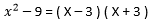# Chapter 1: Polynomials

Definition: A polynomial is an algebraic expression that is a sum of terms, where each term contains only variables with whole number exponents and integer coefficients

Example: The following expressions are all considered polynomials:

When we write a polynomial we follow the convention that says we write the terms in order of descending powers, from left to right.

The following are not polynomials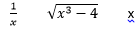A polynomial can have any number of terms (”poly”means ”many” ). We have special names for polynomials that have one, two, or three terms:

Monomial:a monomial has one term (“mono“means “one “ ). The following are monomials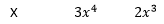Binomial :a binomial has two terms: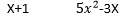Trinomial:a trinomial has three terms: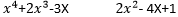DEGREE OF A TERM

The degree of an individual term in a polynomial is the sum of powers of all the variables in that term. We only have to use the plurals in this definition because of the possibility that there may be more than one variable (traditionally denoted by the letter “X“). In that case, the degree will simply be the power of the variable. In a practice, you will most often see polynomials that have only one variable (traditionally denoted by the “X“). In that case, the degree will simply be the power of the variable.

Examples: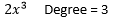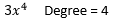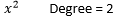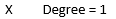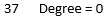DEGREE OF A POLYNOMIAL

The degree of the entire polynomial is the degree of the highest-degree term that it contains, so :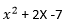is a second-degree trinomial, and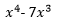is a fourth-degree binomial.

Addition and Substraction of Polynomials : Adding (or subtracting) polynomials is really just an exercise in collecting like terms. For example, if we want to add the polynomial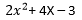to the polynomial 6X-4 We would just put them together and collect like terms:

Notice that the parentheses in the first line are only there to distinguish the two polynomials.Although this is basically just a bookkeeping activity, it can get a little messy when there are many terms. One way to help keep things straight is use the column format for addition, keeping like terms lined up in columns:

This method is particularly helpful in the case of subtraction,because it is too easy to make a mistake distributing the minus sign when you write it all in one row.

MULTIPLICATION OF POLYNOMIALS

• The general rule is that each term in the first factor has to multiply each term in the other factor
• The number of products you get has to be the number of terms in the first factor times the number of terms in the second factor. For example, a binomial times a binomial gives four products, while a binomial times a trinomial gives six products.
• Be very careful and methodical to avoid missing any terms
• After the multiplication is complete  you can try to collect like terms to simplify the result

EXAMPLE: PRODUCT OF A BINOMIAL AND TRIOMIAL

SPECIAL PRODUCTS OF BINOMIAL

Some products occur so frequently in algebra that is advantageous to be able to recognize them by sight. This will be particularly useful when we talk about factoring.

In the following examples the special products of binomials are multiplied out using the FOIL method, and then simplified

DIFFERENCE OF TWO SQUARES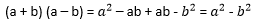SQUARING A BINOMIAL

What you should to be able to recognize by sight are these three formulas: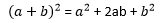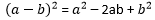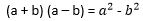You should be able to recognize these products both ways. That is, if you see the left side you should think of the right side, and if you see the right side you should think of the left side.

FACTORING POLYNOMIALS

Factoring a polynomial is the opposite process of multiplying polynomials. Recall that when we factor a number, we are looking for prime that multiply together to give the number, for example :

9= 3×3 ,   12= 4×3,    27= 3x3x3

When we factor a polynomial, we are looking for simpler polynomials that can be multiplied together to give us the polynomial that we started with. You might want to review multiplying polynomials if you are not completely clear on how that works.

When we factor a polynomial that have integer coefficients and constants.

SIMPLEST CASE: REMOVING COMMON FACTORS

The simplest type of factoring is when there is a factor common to every term. In that case, you can factor out that common factor. What are you doing is using the distributive law in reverse you are sort of un-distributing the factor.

Recall that the distributive law says

a(b + c) = ab + ac

Thinking about it in reverse means that if you see ab + ac, you can write it as a a (b + c)

EXAMPLE: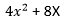Notice that each term has a factor of 2X, so we can rewrite it as: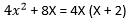DIFFERENCE OF TWO SQUARES

If you see something of the form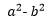, you should remember the formula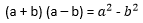EXAMPLE: